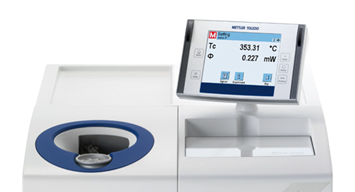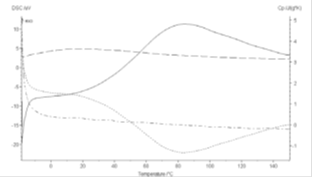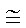## Lesson 6. Measurement of thermal properties of biomaterials

Measurement of thermal properties of biomaterials

Thermal conductivity measurement techniques can be divided into three major groups: a. Steady state Techniques, b. Quasi-steady techniques, c. Transient techniques. The two most used techniques are the Guarded Hotplate and the Heated Probe. It is highly affected by physical properties of the material such as the moisture content, the temperature and structure, such as distribution of component phases, and fiber orientation.

Measurement of Thermal Conductivity

Measurement of thermal conductivity can be done by either steady-state or transient-state methods. There are a number of experimental measurement techniques under each of these two categories.

The advantages of steady-state methods are the simplicity in the mathematical processing of the results, the ease of control of the experimental conditions, and often quite high precision in the results. However, a long time is required for temperature equilibration. The moisture migration and the necessity to prevent heat losses to the environment during this long measurement time are the disadvantages of steady-state methods. In addition, these methods require definite geometry of the sample and relatively large sample size.

On the other hand, the transient methods are faster and more versatile than the steady-state methods and are preferable for extensive experimental measurements. Transient methods are preferred over steady-state methods because of the short experimental duration and minimization of moisture migration problems.

In steady-state methods, two sides of a flat object are maintained at constant but different temperatures and the heat flux through the sample is measured. Steady-state methods are longitudinal heat flow, radial heat flow, heat of vaporization, heat flux, and differential scanning calorimeter methods.

(a) Longitudinal Heat Flow Method: The most common method in this group is guarded hot plate method. This method is suitable mostly for determination of thermal conductivity of dry homogeneous materials in slab forms. It is the most widely used and the most accurate method for the measurement of thermal conductivity of materials, which are poor heat conductors.

In this method, the heat source (T1), the sample, and the heat sink (T2) are placed in contact with each other and with a thermal guard heated electrically. The thermal guard plates are kept at the same temperature as the adjacent surfaces, in a way that no heat leakage takes place from source, sample, or sink boundaries. Thermal conductivity is measured after the sample has reached steady state condition. However, achieving steady-state conditions may take several hours. It is assumed that all of the measured heat input is transferred across the sample. The thermal conductivity is calculated by measuring the amount of heat input required to maintain the unidirectional steady-state temperature profile across the test sample.

(b) Radial Heat Flow Methods. These methods are suitable mostly for loose, powdered, or granular materials.

(i) Concentric Cylinder Method: In this method, the sample is placed between two concentric cylinders. This method is preferable for liquid samples. The heater is usually located at the outer cylinder. A coolant fluid flows through the inner cylinder. The heat that the coolant absorbed is assumed to be equal to the heat transferred through the sample. Thermal conductivity can be calculated from the unidirectional radial steady-state heat transfer equation as

The length-to-diameter ratio of the cylinder must allow the radial heat flow assumption. End guard heaters may be used to minimize the error due to axial heat flow.

(ii) Concentric Cylinder Comparative Method: This method uses a central heater followed by a cylindrical sample and a cylindrical standard. The temperatures T1 and T2 at radii r1 and r2 of the sample, respectively, and temperature T3 and T4 at radii r3 and r4 of the standard, respectively, are measured. Assuming radial heat flow, the thermal conductivity can be determined

Sphere with Central Heating Source. In this method, the sample is placed between the central heater which has a radius r1 and the outer radius of sphere, r2. The sample completely encloses the heating source so that end losses are eliminated. Assuming that the inner and outer surfaces of the sample are T1 and T2, respectively, after the steady state has been established heat flow will essentially be radial thermal conductivity can be determined as:

This is the most sensitive method among the steady-state methods because the error due to heat losses can be practically eliminated. However, it cannot be widely used because of the difficulty in obtaining suitably shaped food samples. This method has been used mainly for granular materials. Samples should be filled in a vacuum environment because air bubbles trapped inside the sphere could increase contact resistance.

(c) Heat of Vaporization Method: In this method, a small test sample is put between two silver plates, one of which is in contact with a liquid A at its boiling point and the other one is in contact with liquid B. Heat transferred through the sample vaporizes some of the liquid B, which has a lower boiling point. Since the time necessary to vaporize a unit mass of liquid B is known, the thermal conductivity of the sample is calculated:

(d) Heat Flux Method: The heat flow meter is a device for measuring heat flux. In this technique, a heat flux sensor is attached to the inner surface of the wall with a very thin layer of high thermal conductivity adhesive. A temperature difference of 5 to 7◦C is maintained within the system and the thermal conductivity is evaluated at the arithmetic mean temperature.

(e) Differential Scanning Calorimeter (DSC): An attachment to a differential scanning calorimeter was designed to measure thermal conductivity of foods. The sample of uniform cross section (possibly cylindrical) is placed in the sample pan, the opposite end of which is in contact with a heat sink at constant temperature. Initially, the sample is maintained at a constant temperature. At a predetermined time, the pan temperature is immediately increased to a predetermined higher value. A new steady state is reached in a few minutes and the heat flow into the DSC pan levels off. The difference in heat flow between the two states is recorded from the thermogram. Then, thermal conductivity of the sample can be calculated using Fourier’s heat conduction equation:

where L is the sample thickness, A is the cross-sectional area,

T1 is the initial temperature difference, and T2 is the final temperature difference.

This method is simple and suitable for small size samples, for both low- and high-moisture foods. Time to achieve the new steady state is small enough (10 to 15 min) to prevent moisture migration since the sample is small. This approach may be modified to measure thermal conductivity as a function of temperature by using small thermal perturbations. However, measurement of thermal conductivity under ultrahigh temperature (UHT) conditions may require extensive equipment modification. Thus, it is more expensive than either the line heat source or modified Fitch methods, which are the most commonly used unsteady-state thermal conductivity measurement methods in food systems.

Using D.S.C based on steady state method.

$k =\left({{L\over {\Delta Q}}}\right)\left( {{A\over{\Delta T_2 -\Delta T_1}}}\right)$                  L= sample length,$\Delta Q$=difference in energy
Required to maintain pan temperature (W)

A= sample area perpendicular to flow (m2)

$\Delta T_2=$Final temperature difference between DSC heating pan & sample (k)

$\Delta T_1=$Initial temperature difference between DSC heating pan & sample (k)

Assumption

Good contact between DSC & sample

Negligible heat loss from radial direction

No thermal gradient in the heat sink

The most important transient methods are the thermal conductivity probe method, transient hot wire method, modified Fitch method, point heat source method, and comparative method.

(a) Thermal Conductivity Probe Method: This method is the most popular method for determining thermal conductivity of food materials because of its relative simplicity and speed of measurement. In addition, this method requires relatively small sample sizes. On the other hand, it requires a fairly sophisticated data acquisition system. In this method, a constant heat source is applied to an infinite solid along a line with infinitesimal diameter, such as a thin resistant wire. The electrical wire must have a low resistance so that the voltage drop across it is negligible compared to the voltage drop across the heater. For measurement of thermal conductivity, the container is filled with sample and the line heat source probe is inserted at the center of the container. The container is placed in a constant temperature bath and equilibrated at room temperature. After the initial temperature is recorded, the probe heater is activated and heated at a constant rate of energy input. Then, the time versus temperature adjacent to the line heat source is recorded.

(b) Transient Hot Wire Method: This method involves a thin heater wire similar to the line heat source method. However, in this case, the hot wire is located at the interface between the sample and a reference of known thermal conductivity. The heater and temperature sensor in the hot wire thermal conductivity apparatus consist of a single wire that is exposed to the material, while in the thermal conductivity probe there are separate wires that are usually sealed in a tube. When electrical power is applied to the heater wire, the temperature rise T at a point located on the interface between the two materials at a distance x from the heater wire may are calculated.

(c ) Modified Fitch Method:. One of the most common transient methods used to measure the thermal conductivity of low conductivity materials is the Fitch method. The Fitch method consists of a heat source or a sink in the form of a vessel filled with constant temperature liquid, and a sink or a source in the form of a copper plug insulated on all sides except one face through which heat transfer occurs. The sample is sandwiched between the vessel and the open face of the plug. Then, the temperature of the plug varies with time depending on the heat flow rate through the sample. Copper may be considered as lumped system since its thermal conductivity is high enough, and its temperature history may be used together with its mass and physical properties for calculation of the sample thermal conductivity.

(d) Point Heat Source Method: This method involves a point heat source, which is heated for a period of time followed by monitoring of its temperature as the heat dissipates through the sample. The typical device used for this purpose is a thermistor that serves as both a heating element and a temperature sensor.

(e) Comparative Method: The comparative method is simple and a range of sample sizes can be handled via this method. Overall thermal conductivity measurement is possible rather than local measurement. Therefore, it is suitable for porous foods such as cakes. This method involves cooling of two spheres side by side in a well stirred ice/water bath. One sphere contains the sample and the other contains a reference of known thermal conductivity. The thermal conductivity of the sample is calculated from the time–temperature data of the cooling spheres. It is based on the analytical solution for the center temperature of a sphere being cooled with convection boundary conditions.

Thermal conductivity measurement:-

Thermal conductivity measurement of food grains varies from 0.3 to 0.6 kcal/m hr°C, for bulk is about 0.10 to 0.15 kcal/ m hr°C, which is due to presence of air space in it. Thermal conductivity of air is 0.02 kcal/ m hr°C. The thermal conductivity is measured with

For wheat,

k= 0.060+0.002m kcal/ m hr°C         (for m.c between 10% to 20%)

k= thermal conductivity

m= m.c(% db)

For water = 0.5 to 0.6 w/m.k

Specific heat & Measurement:-

It could be the seam of specific heat of bone dry matter & of its moisture content.

$C_p=\left({{m\over{100}}}\right)C_W+\left({{{100 - m}\over {100}}}\right)C_d$   For M.C above 8% only

Or $C_p=\left({{m\over{100}}}\right)+\left({{{100-m}\over{100}}}\right)C_d$   kcal/kg°C

Cd= Sp. Of bone dry material, Cw of water = kcal

m= m.c content in wet basis

Sp.heat  of bone dry grain varies between 0.35-0.45 Kcal/kg°C

Mixture method:-

Most widely used for measuring Sp. heat. In this method, the specimen of a union mass temperature is placed in a calorimeter of a known sp. heat containing water or a liquid with known temperature & mass.

One unknown sp. heat of the specimen is then computed using a heat balance between the heat gained by or lost by the water or liquid & calorimeter & the heat cost or gained by the specimen.

ms cp(Tem - T0C)=$c_{pw}(m_w+w)(T_{oc}-T_{em})$
cp= {{{\rm{c}}_{{\rm{pw}}}(m_w  + w)(T_{oc}-T_{em})}\over{m_s (T_{em}-T_{ls} )}}\]
cp= Sp.heat of sample in temperature range of $T_{oc} toT_{em}$(J/ng k)

cpw= average heat of water in the temperature $T_{oc} toT_{em}$

mw = man of water(kg)

ms = man of sample(kg)

W= water equivalent of the system (kg)

Toc= Initial temperature of calorimeter

Tem=Final equilibrium temperature of the mixture

Tes=Initial temperature of sample

The main source of the temperature is thermal leakage from the calorimeter even though losses are negligible.

Specific heat measurement by DSC:

To find out the specific heat of the food sample usually a reference sample of nown specific heat is carried out prior to running the test for the food sample. The reference samples are usually a sapphire disc or distilled water. The sample of uniform cross section (possibly cylindrical) is placed in the sample pan, the opposite end of which is in contact with a heat sink at constant temperature. Initially, the sample is maintained at a constant temperature. At a predetermined time, the pan temperature is immediately increased to a predetermined higher value. A new steady state is reached in a few minutes and the heat flow into the DSC pan levels off. The difference in heat flow between the two states is recorded from the thermogram. The two curves (one for the reference sample) and the food sample thermogram is then compared to find out the specific heat of the sample.Figure of DSC (a) (source: Mettler Toledo) and thermogram(b) source: Mohapatra & Bal, 2003)

Calculations Sp.heat measuring problems

Two compartment calorimeter method

Comparision calorimeter method

$\left({{{dQ}\over{dt}}}\right)_A=\left({C_{PA}m_A }\right)+\left({C_{pw}m_w }\right){{dT}\over {\Delta t_A }}$
$\left({{{\Delta Q}\over {\Delta t}}}\right)_B=\left({C_{PB} m_B+C_{ps} m_s }\right){{\Delta t}\over{dt_B }}$

Q =$\left({m_{cp}\Delta T}\right)_{sample}+\left({m_{cp}\Delta T}\right)_{Conyainer}+\left({m_{cp}\Delta T}\right)_{chamber}$
When no heat or moisture transfer through the chamber is required

A measured quantity of heat is added by heating a wire buried in the bulk of the contains placed in chamber.

${{DifferntialScanningcalorimeter}\over{Sphereasfunctionoftemperature}}$
${{dQ}\over{dt}}=$ heat flow rate w(J/s)$C_p={\raise0.7ex\hbox{{\left({{{dQ}\over{dt}}}\right)}}\!\mathord{\left/{\vphantom{{\left({{{dQ}\over{dt}}}\right)}{m_s }}}\right.\kern-\nulldelimiterspace}\!\lower0.7ex\hbox{{m_s}}}\left({{{dT}\over{dt}}}\right)$
${{dT}\over{dt}}=$
heating rate k/s or c/s , ms=mass/hg

Thermal properties of food constituent at approximately 20°C

 Component Sp. heat kJ/kg°C Thermal conductivity W/m °C Water 4.18 0.60 Carbohydrate 1.42 0.58 Protein 1.55 0.20 Fat 1.67 0.18 Air 0.96 0.25 Ice 2.09(-2°C) -2.4(below °C) Steam 2.01(110°C) - Inorganic minerals0.84 - Metals 0.05-1.0 50-400